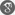##### Cite as:
Maamar Andasmas, Benharrat Belaidi, Growth and Zeros of Meromorphic Solutions to Second-Order Linear Differential Equations, Int. J. Anal. Appl., 11 (1) (2016), 11-18.

#### Abstract

The main purpose of this article is to investigate the growth of meromorphic solutions to homogeneous and non-homogeneous second order linear differential equations f00+Af0+Bf = F, where A(z), B (z) and F (z) are meromorphic functions with finite order having only finitely many poles. We show that, if there exist a positive constants σ > 0, α > 0 such that |A(z)| ≥ eα|z|σ as |z| → +∞, z ∈ H, where dens{|z| : z ∈ H} > 0 and ρ = max{ρ(B), ρ(F)} < σ, then every transcendental meromorphic solution f has an infinite order. Further, we give some estimates of their hyper-order, exponent and hyper-exponent of convergence of distinct zeros.

#### References

1. M. Andasmas and B. Bela¨ıdi, On the growth and fixed points of meromorphic solutions of second order non-homogeneous linear differential equations, Int. J. Math. Comput. 18(2013), no. 1, 28-45.2. B. Bela¨ıdi, Estimation of the hyper-order of entire solutions of complex linear ordinary differential equations whose coefficients are entire functions, Electron. J. Qual. Theory Differ. Equ. 2002 (2002), Article ID 5.3. B. Bela¨ıdi, Growth of solutions of certain non-homogeneous linear differential equations with entire coefficients, JIPAM. J. Inequal. Pure Appl. Math., 5 (2004), no. 2, Article ID 40.4. A. Besicovitch, On integral functions of order < 1, Math. Ann. 97 (1927), no. 1, 677-695.5. Z. X. Chen, Zeros of meromorphic solutions of higher order linear differential equations, Analysis, 14 (1994), no. 4, 425–438.6. Z. X. Chen, The zero, pole and order of meromorphic solutions of differential equations with meromorphic coefficients, Kodai Math. J., 19 (1996), no. 3, 341–354.7. Z. X. Chen and C. C. Yang, Some further results on the zeros and growths of entire solutions of second order linear differential equations, Kodai Math. J., 22 (1999), no. 2, 273–285.8. Y. Chen, Estimates of the zeros and growths of meromorphic solutions of homogeneous and non-homogeneous second order linear differential equations, Math. Appl. (Wuhan) 23 (2010), no. 1, 18-26.9. G. G. Gundersen, Estimates for the logarithmic derivative of a meromorphic function, plus similar estimates, J. London Math. Soc. 37 (1988), no. 1, 88-104.10. G. G. Gundersen, Finite order solutions of second order linear differential equations, Trans. Amer. Math. Soc. 305 (1988), no. 1, 415-429.11. W. K. Hayman, Meromorphic Functions, Clarendon Press, Oxford, 1964.12. S. Hellerstein, J. Miles, J. Rossi, On the growth of solutions of f00+ gf0+ hf = 0, Trans. Amer. Math. Soc., 324 (1991), no. 2, 693–706.13. K. H. Kwon, On the growth of entire functions satisfying second order linear differential equations, Bull. Korean Math. Soc., 33 (1996), no. 3, 487-496.14. I. Laine, Nevanlinna theory and complex differential equations, de Gruyter Studies in Mathematics, 15. Walter de Gruyter & Co., Berlin, 1993.15. J. Wang, I. Laine, Growth of solutions of nonhomogeneous linear differential equations, Abstr. Appl. Anal. 2009 (2009), Article ID 363927.16. C. C. Yang and H. X. Yi, Uniqueness theory of meromorphic functions, Mathematics and its Applications, 557. Kluwer Academic Publishers Group, Dordrecht, 2003.